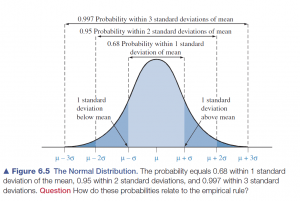19
Aug 16

## The pearls of AP Statistics 21Turn a boring piece of theory into a creative exercise

Here is Figure 6.5 from Agresti and Franklin:I say: why make students memorize this? Why give it as another axiom when they can deduce this themselves? I do it in two steps, to separate the logic from arithmetics. First, I ask two questions: 1) If you have a symmetric bell-shaped distribution and you know the area of the left tail, what will be the area of the right tail symmetric to it? 2) With the same distribution and tails, how much will be the area between the tails if you know the area of one tail? I didn't see a single student who wouldn't be able to answer these questions. Then I tell the students to look up the tail area in the statistical table in Appendix A and find the areas in Figure 6.5. Initially this can be done for the standard normal (μ=0, σ=1) and then generalized for other normals.

Certainly, this takes more time than just giving a ready recipe, but who said the shortest way is the best? By the way, this exercise satisfies the requirements for exercises used for team competitions.

Wolfram Alpha is a good free resource about anything related to Math (if you have access to the Internet). In particular, if you want your students to visualize what happens when σ changes, tell them to enter the command

###### Plot[Evaluate@Table[PDF[NormalDistribution[0, \[Sigma]], x], {\[Sigma], {.75, 1, 2}}], {x, -6, 6}, Filling -> Axis]

Similarly, to see the effect of changing μ, they can enter